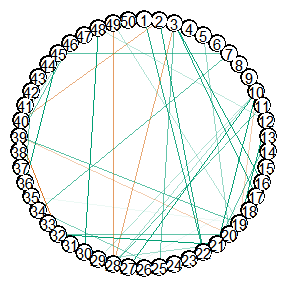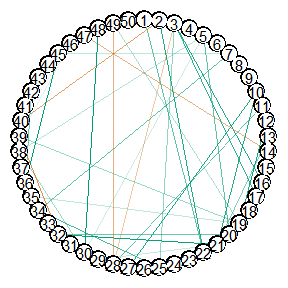# Introduction

GGMncv is set up for low-dimensional settings, that is, when the number of observations ($$n$$) is much greater than the number of nodes ($$p$$). This is perhaps not typical in the Gaussian graphical modeling literature, and is a direct result of my (the author of GGMncv) field encountering low-dimensional data most often (see for example Williams et al. 2019; Williams and Rast 2020). As a result, the defaults are honed in for low-dimensional data!

Of course, GGMncv can readily be used for high-dimensional data. In what follows, I highlight several issues that may arise, in addition to solutions to overcome those issues.

# Failure Altogether

By default, GGMncv uses the sample based (inverse) covariance matrix for the initial values, which is needed for employing nonconvex regularization. When $$p > n$$, GGMncv will produce an error because the sample based (inverse) covariance matrix cannot be inverted in this situation.

For example, notice the error when running the following code:

library(GGMncv)

# p > n
set.seed(2)
main <- gen_net(p = 50,
edge_prob = 0.05)

set.seed(2)
y <- MASS::mvrnorm(n = 49,
mu = rep(0, 50),
Sigma = main$cors) fit <- ggmncv(cor(y), n = nrow(y)) #> Error in solve.default(R): system is computationally singular: reciprocal condition number = 3.39775e-18 ## Solution The solution is to provide an function for the initial matrix. To this end, GGMncv includes the function lediot_wolf which is a shrinkage estimator (Ledoit and Wolf 2004). It is important to note that any function can be used, so long as it return the inverse correlation matrix. fit <- ggmncv(cor(y), n = nrow(y), penalty = "atan", progress = FALSE, initial = ledoit_wolf, Y = y) Notice the Y = y, which is used internally to pass additional arguments via ... to the function provided in initial. The conditional dependence structure can then be plotted with plot(get_graph(fit), node_size = 5)Here is an example of providing a function. initial_ggmncv <- function(y, ...){ Rinv <- corpcor::invcor.shrink(y, verbose = FALSE) return(Rinv) } fit2 <- ggmncv(cor(y), n = nrow(y), penalty = "atan", progress = FALSE, initial = initial_ggmncv, y = y) plot(get_graph(fit2), node_size = 5)Perhaps it is of interest to compare performance, given that different initial values were used. # ledoit and wolf score_binary(estimate = fit$adj,
true = main$adj, model_name = "lw") #> measure score model_name #> 1 specificity 0.9750859 lw #> 2 sensitivity 0.2459016 lw #> 3 precision 0.3409091 lw #> 4 recall 0.2459016 lw #> 5 f1_score 0.2857143 lw #> 6 mcc 0.2583199 lw # Shaffer and strimmer score_binary(estimate = fit2$adj,
true = main$adj, model_name = "ss") #> measure score model_name #> 1 specificity 0.9759450 ss #> 2 sensitivity 0.2459016 ss #> 3 precision 0.3488372 ss #> 4 recall 0.2459016 ss #> 5 f1_score 0.2884615 ss #> 6 mcc 0.2622113 ss # Works but Bad Performance Perhaps a trickier situation is when the covariance matrix can be inverted, but it is still ill-conditioned. This can occur when $$p$$ approaches but does not exceed $$n$$. Here performance can be very bad. # p -> n main <- gen_net(p = 50, edge_prob = 0.05) y <- MASS::mvrnorm(n = 60, mu = rep(0, 50), Sigma = main$cors)

fit <- ggmncv(cor(y), n = nrow(y),
penalty = "atan",
progress = FALSE)

score_binary(estimate = fit$adj, true = main$adj)
#>       measure       score
#> 1 specificity  0.07302405
#> 2 sensitivity  0.88524590
#> 3   precision  0.04766108
#> 4      recall  0.88524590
#> 5    f1_score  0.09045226
#> 6         mcc -0.03444145

This is extremely problematic because there was no error, and the performance was terrible (note: 1 - specificity = the false positive rate).

## Solution

One solution is again to provide a function to initial.

fit <- ggmncv(cor(y), n = nrow(y),
progress = FALSE,
penalty = "atan",
initial = ledoit_wolf, Y = y)

score_binary(estimate = fit$adj, true = main$adj)
#>       measure     score
#> 1 specificity 0.9725086
#> 2 sensitivity 0.2622951
#> 3   precision 0.3333333
#> 4      recall 0.2622951
#> 5    f1_score 0.2935780
#> 6         mcc 0.2632312

An additional solution is to use $$L_1$$-regularization, i.e.,

fit_l1 <- ggmncv(cor(y), n = nrow(y),
progress = FALSE,
penalty = "lasso")

score_binary(estimate = fit_l1$adj, true = main$adj)
#>       measure     score
#> 1 specificity 0.9914089
#> 2 sensitivity 0.1311475
#> 3   precision 0.4444444
#> 4      recall 0.1311475
#> 5    f1_score 0.2025316
#> 6         mcc 0.2215582

A quick comparison of KL-divergence

# atan
kl_mvn(true = solve(main$cors), estimate = fit$Theta)
#>  1.539283

# lasso
kl_mvn(true = solve(main$cors), estimate = fit_l1$Theta)
#>  2.374752